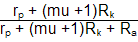Optimal Cathode Resistor Values     The previous formulas were based on using grid bias to set the idle current. Adding a cathode bias resistor complicates matters, as the voltage across the cathode resistor must first be subtracted from the B+ voltage before using the above formulas, but we don't know the cathode resistor's value will be until we know the idle current, which we need the voltage drop across the cathode resistor to determine. If you think this circular, you are right. Fortunately, If the cathode resistor is bypassed, the following formula yields the approximate value for the cathode resistor:Rk = (Ra + rp) / (mu + 1)When the cathode resistor is not bypassed, the following formula yields the greatest symmetrical voltage swing:Rk = (Ra + rp) / (mu - 1)These formulas set the cathode resistor's value to yield the idle current that delivers the greatest symmetrical plate voltage swing by splitting the maximum current draw in half.     If you are bothered by there being two formulas, when the same valued cathode resistor is used, with the same voltage drop across it, considered this: the voltage drop is the same only at idle. When the cathode resistor is bypassed, the voltage drop is fixed for all audio frequencies, but when un-bypassed, the cathode resistor's voltage drop traces the input voltage, so that at one extreme, its voltage drop will equal 0 volts and at the other, twice the idle voltage drop. Output Impedance       Determining the grounded cathode amplifier's output impedance is easy enough, when grid bias or a bypassed cathode resistor are used, as the resistor and the triode's rp in parallel gives the output impedance:          Zo = rp || RaWith an un-bypassed cathode resistor, the formula becomes longer:          Zo = (rp + [mu +1]Rk) || Ra
 PSRR      PSRR stand for "Power Supply Rejection Ratio" and it is usually expressed in decibels, dBs. As you might expect, there are two sets of formulas once again. When grid bias or a bypassed cathode resistor are used:      PSRR = 20Log[rp/(rp + Ra )]With an un-bypassed cathode resistor, the formula once again becomes longer:
 PSRR = 20Log()
 Conclusion      A lot of material has been covered here and much of difficult to grasp at first, but after a little time, you will find that ground-cathode amplifier is really as simple as it looks. The best path to follow is to experiment with real tubes or with a computer program (Tube CAD or SPICE, for example) and compare the results with the theory, as knowing when the theory begins to break down is where art takes over from science                                   //JRB